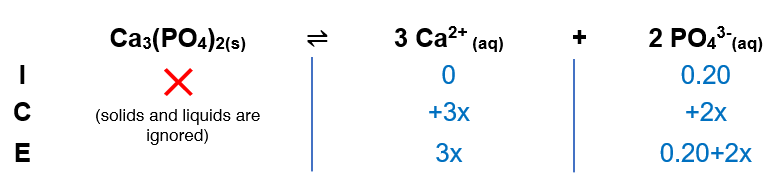# Problem: Calculate the solubility of solid Ca 3(PO4)2 (Ksp = 1.3 X 10 -32) in a 0.20-M Na 3PO4 solution.

###### FREE Expert Solution

Ca3(PO4)2 (s) ⇋ 3 Ca2+ (aq) + 2 PO43- (aq)

(1) Construct an ICE chart(2) Calculate x

91% (50 ratings)###### Problem Details

Calculate the solubility of solid Ca 3(PO4)2 (Ksp = 1.3 X 10 -32) in a 0.20-M Na 3PO4 solution.